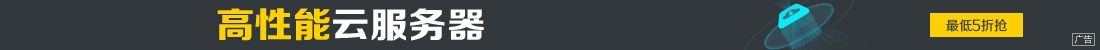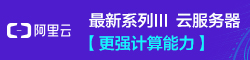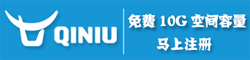# python通过函数属性实现全局变量的方法

••python的函数可以定义属性，而且是全局的，这个非常好用，例如用于数字累加，你不用专门去定义一个全局变量，使用函数的属性即可。

```def add(x=1):
try:
except AttributeError:
class Ax(object):
def __init__(self, val=0):
self.sum = val

def __call__(self, x=1):
self.sum += x
return self.sum